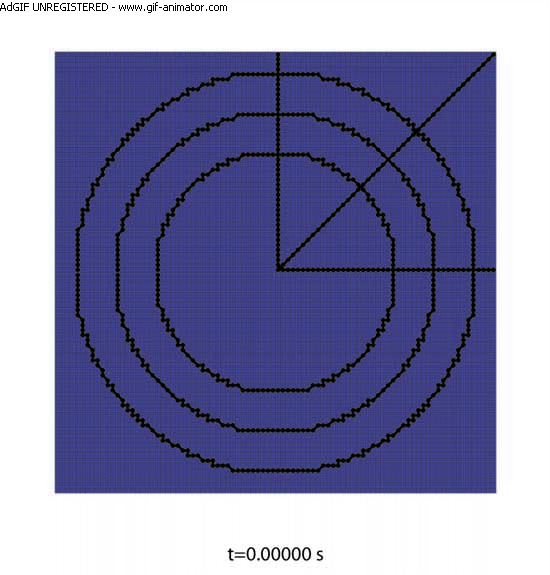# Verification Research: MMS vortex ring simulation

Below are shown comparisons of the exact and numerical solution for the vortex ring problem on a square domain.Motion of the boundary is a visual indication of error, which might seem to be very large until you find out that the above numerical solution was the result of repairing the following entirely unacceptable numerical solution:Quantitative measures of accuracy and convergence are published in